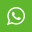## Material Content for PROGRAMMING AND PROBLEM SOLVING THROUGH C LANGUAGE January 2015

1. Each question below gives a multiple choice of answers. Choose the most appropriate one and enter in the “OMR” answer sheet supplied with the question paper, following instructions therein. (1x10)

1.1 The two type of file structures existing in VSAM file are

A) Key sequenced structures, entry sequenced structures

B) Key sequenced structure, exit sequenced structures

C) Entry sequenced structures, exit sequenced structures

D) None of the above

1.2 In a multilist organisation

A) records that have an equivalent value for a given secondary index item are linked together to form a list

B) records are loaded in ordered sequence defined by collating sequence by content of key

C) records are directly accessed by records key field

D) none of the above

1.3 *ptr ++ is equivalent to

A) ptr++

B) *ptr

C) ++ptr

D) ++*ptr

1.4 Declaration int *(*p) int(*a) is

A) A pointer to function that accept an integer argument and return an integer

B) A pointer to a, which returns an integer

C) A pointer to subroutine which returns an integer

D) None of the above

1.5 The output of following program would be

main()

{ printf(“d%d%d%” sizeof(3.14f), sizeof(3.14l);}

IS

A) 4,8,10

B) 8,4,10

C) 18,8,4

D) None of the above

1.6 An example of hierarchical data structure is

A) array

C) tree

D) all of the above

1.7 The do..while looping statement

A) is executed only once if the conditions is true

B) is also known as entry-controlled loop

C) is executed at least once if the conditions is false

D) the unconditional loop

1.8 Which of the following is not a proper storage class in ‘C’?

A) auto

B) dec

C) static

D) extern

1.9 The average number of comparison is sequential search is

A) n**2

B) n(n-1)/2

C) n(n+1)/2

D) (n+1)/2

2. Each statement below is either TRUE or FALSE. Choose the most appropriate one and ENTER in the “OMR” answer sheet supplied with the question paper, following instructions therein. (1x10)

2.1 A command that lets you change one or more fields in a record is look up.

2.2 In relational schema each tuple is divided into fields called relations.

2.3 The best average SORTING behavior is shown by merge sort.

2.4 The data structure that is needed to convert infix notation to prefix notation is graph.

2.5 The best case and worst case time for searching an element using linear search is 1, n.

2.6 Forest is a set of N>=0 disjoint trees, which are obtained when root is removed.

2.7 PERT graph is a graph used to perform PERT calculation.

2.8 Priority queue is a queue that is used to store priority data.

2.9 A symmetric diagraph is a directed graph such that for every edge VW there is reverse edge WV.

2.10 Maximum degree in any vector in a graph with n vertices is n-1.

3. Match words and phrases in column X with the closest related meaning/ word(s)/phrase(s) in column Y. Enter your selection in the “OMR” answer sheet supplied with the question paper, following instructions therein. (1x10)

 QUESTION OPTION 3.1 Null pointer is A. Files that are stored on a direct access medium 3.2 Number of operators required in ?: operators B. 2 bytes 3.3 Null macros is C. manipulator 3.4 A direct access file is D. Number of tracks and number of sectors per track 3.5 Seek time is E. The region over which the variable declaration has effect 3.6 A function that change state object is called F. A pointer which does not point anywhere 3.7 FORMAT command in DOS is used to establish G. Three 3.8 Scope of a variable is H. Contains pointer to itself 3.9 A self referential structure is I. Files that are stored on a random access medium 3.10 Size of a pointer in small memory model is J. A macro that represent null pointer K. Depends upon context L. Time taken by read write head mechanism to position itself over appropriate cylinder M. Two

4. Each statement below has a blank space to fit one of the word(s) or phrase(s) in the list below. Enter your choice in the “OMR” answer sheet supplied with the question paper, following instructions therein. (1x10)

4.1 An example of volatile memory is ________.

4.2 The ________ operator is true only when both the operands are true.

4.3 LINUX is an example of ________.

4.4 By default a real number is treated as ________.

4.5 Header file in which NULL macro is defined is ________.

4.6 Memory that can be allocated in a single call to macro is ________.

4.7 Total number of comparison in bubble sort is ________.

4.8 Arranging pack of cards by picking one by one is an example of ________.

4.9 All pair shortest path problem can be solved by ________.

4.10 Avoid the use of ________ statement in the program.